Notebook

Portfolio Optimization on Quantopian¶

Suppose you've written an algorithm that's developed a signal (for example, a Pipeline Factor) that's predictive of forward returns over some time horizon. You might think that the hard part is over, but you're still left with the daunting task of translating your signal into an algorithm that can turn a profit while also managing risk exposures.

Traditionally in quantitative finance, the solution to the problem of maximizing returns while constraining risk has been to employ some form of Portfolio Optimization, but performing sophisticated optimizations is challenging on today's Quantopian.

Python libraries like scipy.optimize, CVXOPT, and CVXPY (all available on Quantopian today) provide generic tools for solving optimization problems. These libraries are powerful and flexible, but it takes significant expertise to convert the data structures available on Quantopian into the specific formats understood by these libraries.

Algorithm authors who want to perform even simple optimizations spend much of their time to figuring out how to encode conceptually simple ideas like "constrain gross leverage" into complicated matrices that are hard to interpret and hard to debug when something goes wrong.

The open-source Python ecosystem already provides excellent implementations of the algorithms needed to implement a quality portfolio optimization library. What's missing is an interface that conveniently maps domain concepts from finance to the low-level primitives exposed by existing libraries.

Today we're announcing quantopian.experimental.optimize, a suite of new tools designed to make portfolio optimization on Quantopian more accessible to algorithm authors. As the name suggests, all the functions and classes currently available are experimental and should be considered subject to change at any time. We are releasing these APIs early in the development cycle so that we can gather feedback about how to make them as useful as possible to the community when the time comes for a stable release.

NOTE: The optimization API is only available in notebooks for this release. Work is currently ongoing to make optimization available in the backtester. See the Next Steps section at the bottom of this notebook for more details.

What is Portfolio Optimization?¶

Many algorithms written on Quantopian reach a point where they need to solve an optimization problem of the following form:

Given a list of stocks, choose a vector,

$$w$$

of weights in each stock that maximizes (or minimizes) an objective function,

$$F(w)$$

subject to a set of inequality constraints,

\begin{align} C_1(w) &\leq h_1 \\ C_2(w) &\leq h_2 \\ &\dots \\ C_N(w) &\leq h_N \\ \end{align}

Example:¶

An algorithm builds a model that predicts expected returns for a list of stocks. The algorithm wants to allocate a limited amount of capital to those stocks in a way that gives it the greatest possible expected return without placing too big a bet on any single stock.

We can frame this as an optimization problem as follows:

Given an expected returns vector $r = [r_0, r_1, \dots, r_n]$, a maximum single-stock weight $w_{max}$, and a maximum total weight $W_{max}$, find a portfolio weight vector $w = [w_0, w_1, \dots, w_n]$ that solves the following system:

\begin{align} \text{maximize}&& \\ &&r \cdot w \\ \text{subject to}&& \\ &&w_i &\leq w_{max} && 0 \leq i \leq n\\ && \sum_{i=0}^{n}{|w_i|} &\leq W_{max} \end{align}

A more sophisticated algorithm might introduce a more complex objective (e.g. "maximize return with a penalty applied for expected volatility"), or it might introduce more complex constraints (e.g. limit exposure to particular market sectors).

API Overview¶

The optimize module has three major components in this release:

1. calculate_optimal_portfolio, a top-level entrypoint.
2. Objective classes, representing functions to be minimized or maximized by the optimizer.
3. Constraint classes, representing constraints to be enforced by the optimizer.

Lists of the currently available objectives and constraints can be found under optimize.objectives and optimize.constraints, respectively.

To run a portfolio optimization, you call calculate_optimal_portfolio and provide three values:

• An Objective to optimize.
• A list of Constraints to enforce.
• A pandas Series containing weights for the current portfolio. The index of the current portfolio series defines the assets that are allowed in the target portfolio.
In :
import quantopian.experimental.optimize as opt
print opt.calculate_optimal_portfolio.__doc__

    Calculate portfolio weights optimizing an objective subject to constraints.

Parameters
----------
objective : Objective
The objective function to optimize.
constraints : list[Constraint]
List of constraints on the output portfolio.
current_portfolio : pd.Series
A Series containing the current portfolio weights, expressed as
percentages of the portfolio's liquidation value.

The index of current_portfolio defines what assets are available
for the output portfolio. Assets that are under consideration but not
currently held should be provided with a weight of 0.

Returns
-------
optimal_portfolio : pd.Series
A Series indexed like current_portfolio containing new portfolio
weights. Weights should be interpreted in the same way as
current_portfolio.

Raises
------
InfeasibleConstraints
Raised when there is no possible portfolio that satisfies the received
constraints.
UnboundedObjective
Raised when the received constraints are not sufficient to put an upper
(or lower) bound on the calculated portfolio weights.


In :
opt.objectives

Out:
[quantopian.experimental.optimize.MaximizeAlpha,
quantopian.experimental.optimize.TargetPortfolio]
In :
opt.constraints

Out:
[quantopian.experimental.optimize.CannotHold,
quantopian.experimental.optimize.FixedWeight,
quantopian.experimental.optimize.Frozen,
quantopian.experimental.optimize.LongOnly,
quantopian.experimental.optimize.MaxGrossLeverage,
quantopian.experimental.optimize.MaxTurnover,
quantopian.experimental.optimize.NetLeverage,
quantopian.experimental.optimize.NetPartitionExposure,
quantopian.experimental.optimize.Pair,
quantopian.experimental.optimize.PositionConcentration]

Example: Maximize Expected Returns with Leverage and Concentration Constraints¶

Let's look at how we can use the optimize API to solve the example outlined above.

In :
import numpy as np
import pandas as pd

def draw_asset_barplot(weights, title, plot_kwargs=None):
"""Draw a bar plot from a Series with Asset labels."""
return draw_barplot(
weights,
title,
xticks=[asset.symbol for asset in weights.index],
plot_kwargs=plot_kwargs,
)

def draw_barplot(weights, title, xticks=None, plot_kwargs=None):
"""Draw a bar plot from a pd.Series."""

# Draw the plot. Forward plot_kwargs (if provided) as keywords to plot.
axes = weights.plot(kind='bar', rot=0, fontsize=12, **(plot_kwargs or {}))
axes.grid(False, axis='x')

# Set a title.
axes.set_title(title, {'fontsize': 14})

# Set xtick labels, if provided.
if xticks is not None:
axes.set_xticklabels(xticks)

return axes

In :
# Choose a small universe to make the graphs manageable.
universe = symbols(
['AAPL', 'MSFT', 'TWTR', 'BP', 'XOM', 'MCD', 'QSR'],
symbol_reference_date='2016-10-07',
)

def fancy_returns_model(assets):
"""Not actually fancy."""
rng = np.random.RandomState(5)
return pd.Series(index=assets, data=rng.randn(len(assets)))

empty_portfolio = pd.Series(index=universe, data=0.0)
expected_returns = fancy_returns_model(universe)

In :
draw_asset_barplot(expected_returns, 'Expected Returns');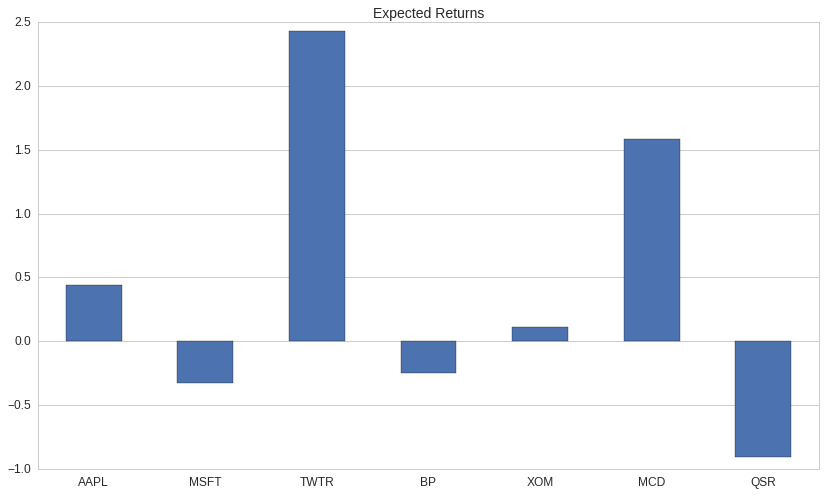Since our goal is to maximize a function directly proportional to asset returns, we'll use opt.MaximizeAlpha as our objective function.

In :
print opt.MaximizeAlpha.__doc__

    Objective that maximizes sum(weights * alphas) for a vector of alphas.

The input vector alphas should contain coefficients such that
alphas[asset] is proportional to the expected return of asset for
the time horizon over which the target portfolio will be held.

In the special case that alphas is an estimate of expected returns for
each asset, this objective simply maximizes the expected return of the
total portfolio.

Parameters
----------
alphas : pd.Series[Asset -> float] or dict[Asset -> float]
Map from assets to alpha coefficients for those assets.

Notes
-----
This objective should almost always be used with a MaxGrossLeverage
constraint, and should usually be used with a PositionConcentration
constraint.

Without a constraint on gross leverage, this objective will raise an error
attempting to allocate an unbounded amount of capital to every asset with a
nonzero alpha.

Without a constraint on individual position size, this objective will
allocate all of its capital in the single asset with the largest expected
return.



The documentation for MaximizeAlpha warns us that we need to put a constraint on the gross leverage of our portfolio or else the optimizer will try to allocate an unbounded amount of capital, so we'll add a MaxGrossLeverage constraint as well.

In :
print opt.MaxGrossLeverage.__doc__

    Constraint on the maximum gross leverage for the portfolio.

Requires that the sum of the absolute values of the portfolio weights be
less than max.

Parameters
----------
max : float
The maximum gross leverage of the portfolio.


In :
def optimal_portfolio_constrained_leverage_only():
"""Calculate the optimal portfolio if we're only constrained by gross leverage."""

# Our objective function.
objective = opt.MaximizeAlpha(expected_returns)

# A list containing our constraints.
constraints = [opt.MaxGrossLeverage(1.0)]

return opt.calculate_optimal_portfolio(objective, constraints, empty_portfolio)

In :
constrained_leverage_pf = optimal_portfolio_constrained_leverage_only()
draw_asset_barplot(constrained_leverage_pf, 'Optimal Portfolio (Constrained Leverage Only)');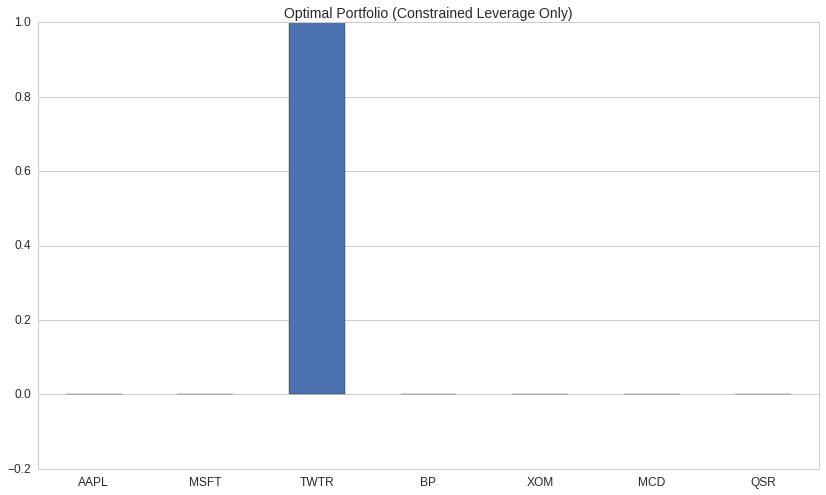With no constraints other than maximizing expected return, the optimizer decided to allocate all of our capital to a single long position in TWTR. While this might be an acceptable portfolio for someone of a gambling disposition, we'd probably rather not put all our eggs in one basket.

We can force the optimizer to diversify our portfolio by adding a PositionConcentration constraint:

In :
print opt.PositionConcentration.__doc__

    Constraint enforcing minimum/maximum position weights.

Parameters
----------
min_weights : pd.Series[Asset -> float] or dict[Asset -> float]
Map from asset to minimum position weight for that asset.
max_weights : pd.Series[Asset -> float] or dict[Asset -> float]
Map from asset to maxium position weight for that asset.
default_min_weight : float, optional
Value to as a lower bound for assets not found in min_weights.
Default is 0.0.
default_max_weight : float, optional
Value to as a lower bound for assets not found in max_weights.
Default is 0.0.

Notes
-----
Negative weight values are interpreted as bounds on the magnitude of short
positions. A minimum weight of 0.0 constrains an asset to be long-only. A
maximum weight of 0.0 constrains an asset to be short-only.

A common special case is to create a PositionConcentration constraint that
applies a shared lower/upper bound to all assets. An alternate constructor,
PositionConcentration.from_constants, provides a simpler API supporting
this use-case.


In :
# Allow 15% of our liquidation value to be in any single short position.
# Allow 30% of our liquidation value to be in any single long position.
# Try changing these parameters to see how they change the results!
MIN_POSITION_WEIGHT = -0.15
MAX_POSITION_WEIGHT = 0.30

def optimal_portfolio_constrained_leverage_and_concentration():
"""Calculate the optimal portfolio if we're constrained by leverage and concentration."""
objective = opt.MaximizeAlpha(expected_returns)
constraints = [
opt.MaxGrossLeverage(1.0),
opt.PositionConcentration.from_constants(min=MIN_POSITION_WEIGHT, max=MAX_POSITION_WEIGHT)
]
return opt.calculate_optimal_portfolio(objective, constraints, empty_portfolio)

In :
constrained_concentration_pf = optimal_portfolio_constrained_leverage_and_concentration()
plot = draw_asset_barplot(
constrained_concentration_pf,
"Optimal Portfolio (Constrained Leverage and Concentration)",
{'ylim': (-MAX_POSITION_WEIGHT - 0.1, MAX_POSITION_WEIGHT + 0.1)},
)
plot.axhline(MIN_POSITION_WEIGHT, color='r', linestyle='dashed')
plot.axhline(MAX_POSITION_WEIGHT, color='r', linestyle='dashed');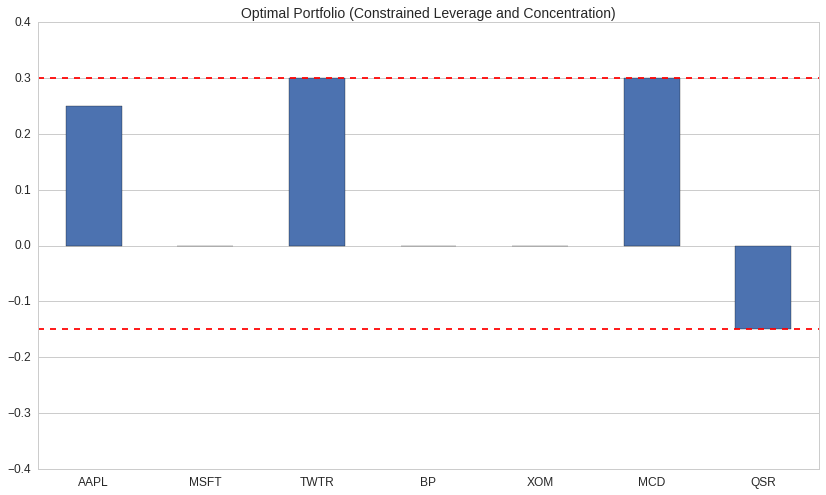With position size constraints, our portfolio is significantly more diverse than before. The optimizer was forced to cap the long allocations to TWTR and GM at 30% of NLV (Net Liquidation Value), and it was forced to cap the short allocations to MCD and BK at 15% NLV. The remaining 10% under the gross leverage cap went to AAPL.

Eyeballing these distributions a bit, we might notice that we're heavily exposed to the technology and food service industries. We can confirm that concern by using Pipeline to grab sector codes for our portfolio.

In :
from quantopian.pipeline import Pipeline
from quantopian.pipeline.classifiers.morningstar import Sector
from quantopian.pipeline.filters import SpecificAssets
from quantopian.research import run_pipeline

def get_sector_names(stocks, date):
"""Get sector codes for a set of stocks for a particular date."""
result = run_pipeline(
Pipeline({'sector': Sector()}, screen=SpecificAssets(stocks)),
start_date=date,
end_date=date
)
# result will have a pd.MultiIndex whose first level just contains date.
# Drop the first level so that we just get assets as our index.
sector_codes = result['sector'].reset_index(level=0, drop=True)

# Convert integral sector codes to human-readable strings by doing a lookup
# from Sector.SECTOR_NAMES, which is a dict mapping codes to strings.
#
# This is purely for readability of the charts below.
# We could proceed with the rest of the notebook using sector codes and it would work just fine.
return sector_codes.map(Sector.SECTOR_NAMES)

In :
sector_names = get_sector_names(universe, '2016-10-07')
sector_names

Out:
Equity(24 [AAPL])              TECHNOLOGY
Equity(4707 [MCD])      CONSUMER_CYCLICAL
Equity(5061 [MSFT])            TECHNOLOGY
Equity(8347 [XOM])                 ENERGY
Equity(19675 [BP])                 ENERGY
Equity(45815 [TWTR])           TECHNOLOGY
Equity(48215 [QSR])     CONSUMER_CYCLICAL
Name: sector, dtype: object
In :
def plot_sector_exposure(pf, sectors):
return draw_barplot(
pf.groupby(sectors).sum(),
'Portfolio Concentration by Sector',
);

plot_sector_exposure(constrained_concentration_pf, sector_names);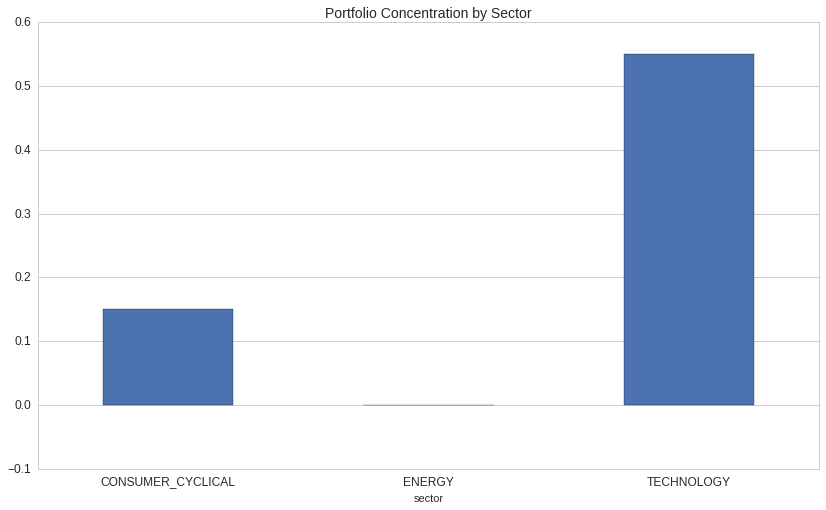As we suspected, we're heavily exposed to the technology sector, and we're moderately exposed to cyclical consumer goods.

We can tell the optimizer to try to build a sector neutral portfolio by adding a NetPartitionExposure constraint:

In :
print opt.NetPartitionExposure.__doc__

    Constraint requiring bounded net exposure to market sub-partitions.

Partitions are specified as a map from (asset -> label). Each unique label
generates a constraint specifying that the sum of the weights of assets
mapped to that label should be approximately zero.

Min/Max partition exposures are specified as maps from (label -> float).

Examples of common partitions are sector, industry, and country.

Parameters
----------
labels : pd.Series[Asset -> object] or dict[Asset -> object]
Map from asset -> partition label.
min_weights : pd.Series[object -> float] or dict[object -> float]
Map from label to minimum net exposure to that label.
max_weights : pd.Series[object -> float] or dict[object -> float]
Map from label to maximum net exposure to that label.

Notes
-----
When this constraint is applied, assets with unknown partition labels will
have their position sizes forced to zero.


In :
ALL_SECTOR_NAMES = Sector.SECTOR_NAMES.values()

def optimal_portfolio_sector_neutral():
"""
Calculate the optimal portfolio if we're constrained by leverage, concentration,
and sector neutrality.
"""
objective = opt.MaximizeAlpha(expected_returns)
constraints = [
opt.MaxGrossLeverage(1.0),
opt.PositionConcentration.from_constants(min=MIN_POSITION_WEIGHT, max=MAX_POSITION_WEIGHT),
opt.NetPartitionExposure(
labels=sector_names,
min_weights={sector: -0.0001 for sector in ALL_SECTOR_NAMES},
max_weights={sector: 0.0001 for sector in ALL_SECTOR_NAMES},
)
]
return opt.calculate_optimal_portfolio(objective, constraints, empty_portfolio)

In :
optimal_sector_neutral_pf = optimal_portfolio_sector_neutral()
plot = draw_asset_barplot(
optimal_sector_neutral_pf,
'Optimal Portfolio (Sector Neutral)',
{'ylim': (-MAX_POSITION_WEIGHT - 0.1, MAX_POSITION_WEIGHT + 0.1)},
)
plot.axhline(MIN_POSITION_WEIGHT, color='r', linestyle='dashed')
plot.axhline(MAX_POSITION_WEIGHT, color='r', linestyle='dashed');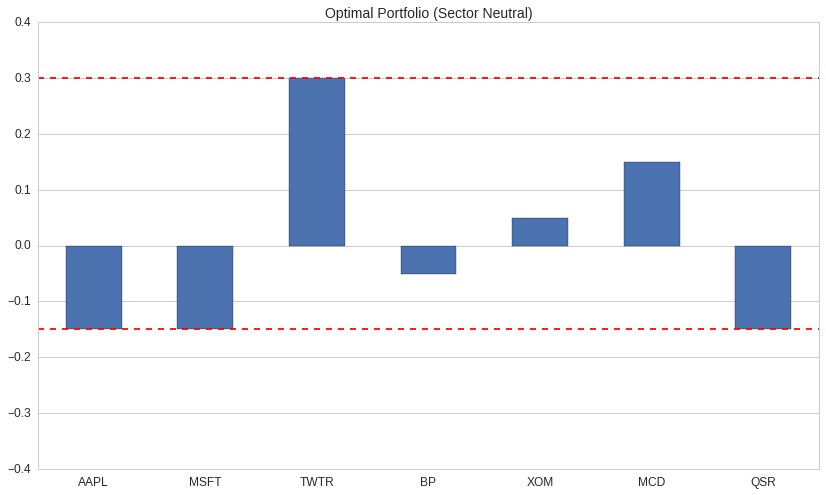In :
plot_sector_exposure(optimal_sector_neutral_pf, sector_names);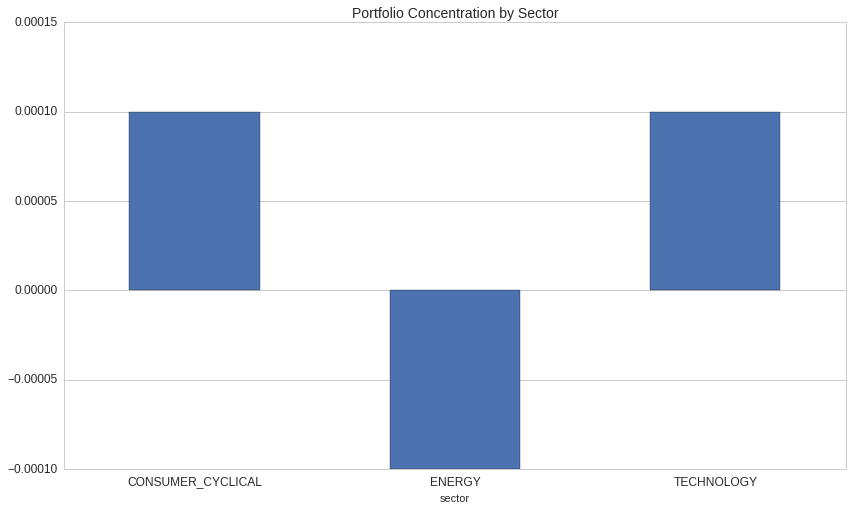With our partition exposure constraint in place, the optimizer chooses to hedge our large TWTR position with shorts in AAPL in MSFT. The optimizer also reigns in the large long in MCD from 30% to 15%, because our 15% short exposure constraint prevents us from getting a equal-sized hedge in QSR, which is the only other CONSUMER_CYCLICAL stock in our universe. That leaves 10% of our capital unallocated, so the optimizer divides it evenly into a pair on BP and XOM.

Example: Minimize Distance from Target Portfolio with Turnover Constraints¶

Many algorithms written on Quantopian today perform stock selection via a process other than explicit returns/alpha-modeling. Pair-trading algorithms, for example, work by identifying pairs of cointegrated stocks and entering into a long position in one half of the pair and a short position in the other half of the pair.

For these existing algorithms (or for new algorithms written in a style other than alpha-modeling), it may not be natural to express portfolio construction in terms of an explicit alpha-maximization problem. Nevertheless, it may still be useful to frame the portfolio construction process as an optimization problem using different objectives and constraints.

Suppose we have an algorithm that trades in four pairs and periodically decides which of the pairs it should allocate capital toward. Via its own internal logic, the algorithm arrives at a "target portfolio" that expresses its ideal holdings. The algorithm wants to trade from its current holdings into its ideal holdings, but it doesn't turn over its entire portfolio at once because doing so may incur excessive transaction costs. The algorithm also wants to ensure that it maintains a net exposure of 0 in all of its pairs.

We can frame this as an optimization problem as follows:

Minimize the distance between the new portfolio and the ideal portfolio, subject to the constraints that:

1. The long components of our pairs have a weights $\geq$ 0.
2. The short components of our pairs have weights $\leq$ 0.
3. The sum of the weights in each of our pairs is 0.
4. The sum of the absolute values of the changes in weight across all stocks is less than our max turnover.

In fancy math language, we can say the following:

Given a vector $W_{current}$ of current portfolio weights, a vector $W_{ideal}$ of ideal portfolio weights, a maximum turnover rate $T_{max}$, and a list of pairs of long/short stocks $P = [(l_1, s_1), (l_2, s_2), \dots, (l_n, s_n)]$, choose a vector $w$ of weights that solves the following system:

\begin{align} \text{minimize}&& \\ &&||w - W_{ideal}||_2 \\ \text{subject to}&& \\ &&w_l &\geq 0&& l \in [l_1, l_2, \dots, l_n] \\ &&w_s &\leq 0&& s \in [s_1, s_2, \dots, s_n] \\ &&w_l + w_s &= 0&& (l, s) \in P \\ &&||w - W_{current}||_1 &\leq T_{max}&& \\ \end{align}

(In the above, $||v||_1$ is the $L^1$ norm of $v$, or the "Taxicab Norm", and $||v||_2$ is the $L^2$ norm of $v$, i.e, the standard Euclidean Norm.)

While the mathematical definition of the problem here is a bit hairy, we can express the problem succintly using the TargetPortfolio objective and the Pair and MaxTurnover constraints.

In :
from itertools import chain

pairs = [
symbols(['AAPL', 'MSFT']),
symbols(['GM', 'F']),
symbols(['MCD', 'QSR']),
symbols(['BP', 'XOM']),
]

# Flatten the list of pairs into a single list for use as an index.
all_pair_stocks = list(chain.from_iterable(pairs))

# Initially allocate half of our capital in the AAPL/MSFT pair and half in the GM/F pair.
initial_pairs_portfolio = pd.Series({
pairs:  0.25,  # AAPL
pairs: -0.25,  # MSFT
pairs:  0.25,  # GM
pairs: -0.25,  # F
}).reindex(
all_pair_stocks,
fill_value=0.0,
)

draw_asset_barplot(initial_pairs_portfolio, 'Initial Pairs Portfolio');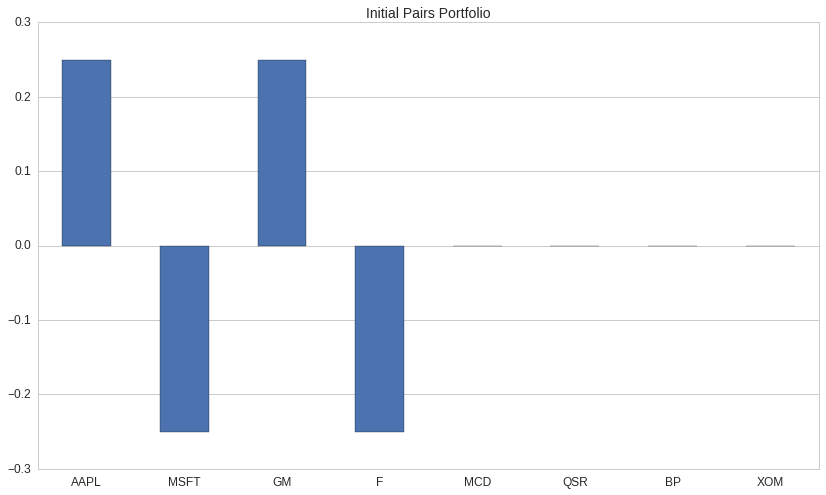Suppose that after some period of time, our algorithm decides that it wants to exit the AAPL/MSFT pair and enter the MCD/QSR and BP/XOM pairs. It might calculate a new target portfolio that's equally-weighted between the three new pairs.

In :
target_pairs_portfolio = pd.Series({
pairs:  0.16666,
pairs: -0.16666,
pairs:  0.16666,
pairs: -0.16666,
pairs:  0.16666,
pairs: -0.16666,
}).reindex(
all_pair_stocks,
fill_value=0.0,
)

draw_asset_barplot(target_pairs_portfolio, 'Target Pairs Portfolio');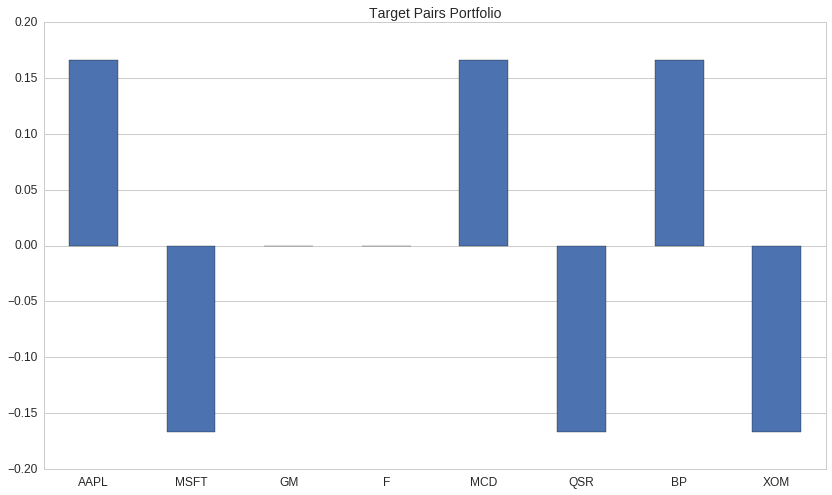If we naively run calculate_target_weights using an unconstrained TargetPortfoio objective, we'll get back our target. But if we supply turnover and pairs constraints, we'll move our capital incrementally out of the current pairs and into the new ones:

In :
print opt.TargetPortfolio.__doc__

    Objective that minimizes the distance from an already-computed portfolio.

Parameters
----------
target_weights : pd.Series[Asset -> float] or dict[Asset -> float]
Map from asset to target percentage of holdings.

A value of 1.0 indicates that 100% of the portfolio's net
liquidation value should be held in the corresponding asset.


In :
print opt.Pair.__doc__

    A constraint representing a pair of inverse-weighted stocks.

Parameters
----------
long : Asset
The asset to long.
short : Asset
The asset to short.
max_net_exposure : float, optional
The maximum allowable net exposure to the pair. Default is 0.00001.


In :
print opt.MaxTurnover.__doc__

    A constraint enforcing a maximum turnover for the optimal portfoio.

Turnover is computed as the sum of the magnitude of the difference in
weights for each asset in the portfolio, i.e., as::

sum(abs(new_weights - old_weights))

Parameters
----------
max : float
The maximum allowable turnover across the whole portfolio.


In :
def migrate_pairs_constrain_turnover(current_pf, target_pf, max_turnover):
"""Calculate a new target portfolio while respecting turnover and pair constraints."""
objective = opt.TargetPortfolio(target_pf)

constraints = [opt.Pair(long, short) for long, short in pairs]
constraints.append(opt.MaxTurnover(max_turnover))

return opt.calculate_optimal_portfolio(
objective=objective,
constraints=constraints,
current_portfolio=current_pf,
)

In :
# Try changing with the MAX_TURNOVER parameter here to see how it affects
# the resulting portfolio!
MAX_TURNOVER = 0.75

migrated_pairs_portfolio = migrate_pairs_constrain_turnover(
current_pf=initial_pairs_portfolio,
target_pf=target_pairs_portfolio,
max_turnover=MAX_TURNOVER,
)

In :
before_and_after_frame = pd.DataFrame(
{
'Initial Weight': initial_pairs_portfolio,
'Migrated Weight': migrated_pairs_portfolio,
'Target Weight': target_pairs_portfolio,
},
# Explicitly set a column order so that the plot below is
# created in the order we want.
columns=['Initial Weight', 'Migrated Weight', 'Target Weight']
)

ax = draw_asset_barplot(
before_and_after_frame,
# The fancy brace syntax here is a format specification.
'Optimal Portfolio: {0:.0f}% Max Turnover '.format(MAX_TURNOVER * 100),
);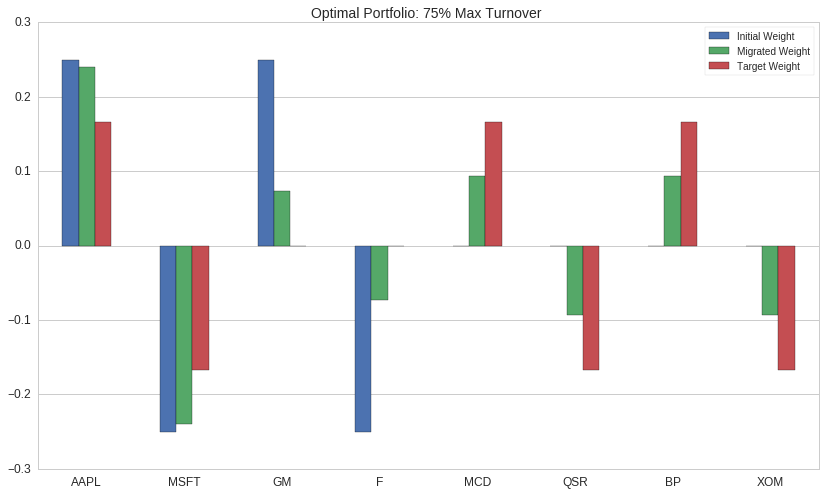Since the optimizer can't reach its target portfolio without violating the MaxTurnover constraint, it attempts to equalize the size of the difference between each stock's output weight and it's target weight.

In :
print "Total Turnover: %f\n" % (migrated_pairs_portfolio - initial_pairs_portfolio).abs().sum()
print "Absolute Difference from Target Weight:\n"
print (migrated_pairs_portfolio - target_pairs_portfolio).abs()

Total Turnover: 0.750000

Absolute Difference from Target Weight:

Equity(24 [AAPL])      0.072915
Equity(5061 [MSFT])    0.072915
Equity(40430 [GM])     0.072915
Equity(2673 [F])       0.072915
Equity(4707 [MCD])     0.072915
Equity(48215 [QSR])    0.072915
Equity(19675 [BP])     0.072915
Equity(8347 [XOM])     0.072915
dtype: float64


Next Steps¶

This notebook provided an overview of the APIs currently available in quantopian.experimental.optimize. There's still a lot of work to do and many questions to answer before we can move beyond experimental status:

• The optimize module is currently only available in the research environment, not in algorithms. What changes are necessary to make optimize available for algorithms? In particular, are there additional APIs that would be valuable just for algorithms? For example, the need to pass in your current portfolio is unnecessary in the context of an algorithm, since the algorithm executor already knows what the current portfolio is. The process of ordering based on the results of an optimization could also be streamlined via new built-ins like an order_target_portfolio method.

• In the example above, we had to "manually" build a sector neutral constraint by using Pipeline to query for sector codes, formatting the results, and passing them to NetPartitionExposure. Common applications like this could be simplified with "data-aware" constraints that could fetch data at optimization time. A built-in SectorNeutral constraint, for example, might internally use Pipeline machinery to fetch morningstar sector codes without requiring any additional input from users. Are there other examples of built-in constraints that could benefit from such a treatment? How should data-aware constraints work in notebooks where there isn't an implicit simulation date?

• How can the existing Constraints and Objectives be simplified or improved?

• What new Constraints should be implemented?
• What new Objectives should be implemented?

With help from the Quantopian community, we hope to answer these questions in the coming weeks and months. I'm excited about the possibilities that new APIs create for algorithm authors, and I look forward to hearing feedback and seeing what the community builds with these tools.